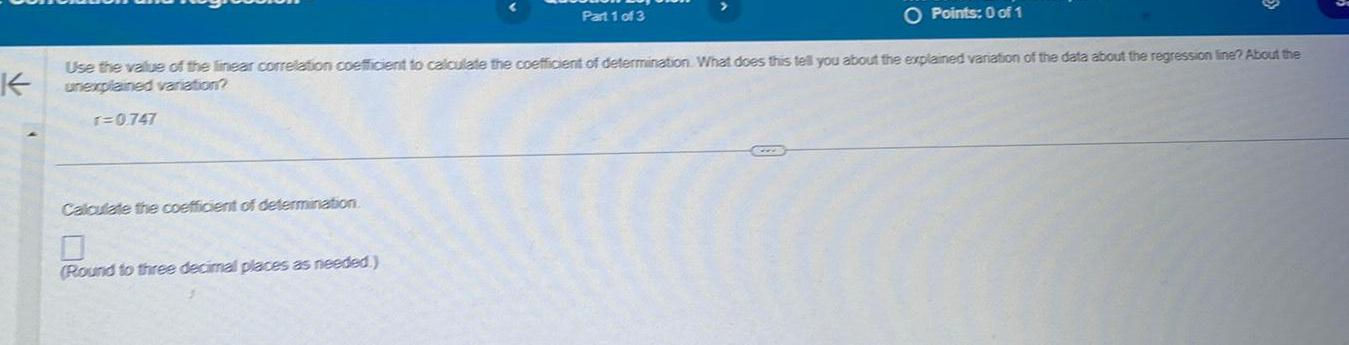Question:

# K O Points 0 of 1 Use the value of the linear correlation

Last updated: 7/29/2023K O Points 0 of 1 Use the value of the linear correlation coefficient to calculate the coefficient of determination What does this tell you about the explained variation of the data about the regression line About the unexplained variation r 0 747 Calculate the coefficient of determination Part 1 of 3 Round to three decimal places as needed